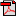# Lesson 1: What Is Energy?

## Activities

### Activity 1: Forms of Energy

There are many different forms of energy, as described in the reading. Select one of the following options.
For this activity your child will select one of the following options. For Option 1 he will illustrate each form of energy, and for Option 2 he will think of a way to demonstrate each form of energy.

#### Option 1: Picture of Energy

Create your own graphic or illustration for each form of energy. Your images should be different from those found in the book.Student Activity PageStudent Activity PageStudent Activity Page
For this option check to see that your child has accurately illustrated each form of energy. Ask him to explain how he came up with his ideas. His images should be different than the ones in the book.

#### Option 2: Demonstration of Energy

Think of a way to demonstrate each form of energy so that a person can better understand and remember it. Make a list of items you would need for your demonstration.Student Activity PageStudent Activity PageStudent Activity Page
Review your child's demonstration ideas. If he would like to do any of the demonstrations, find a time during the day for him to do so. Provide feedback on the accuracy of his demonstrations.

### Activity 2: Energy and Work

Materials: calculator*
When energy causes change, we call it work. In order for work to be accomplished, there must be a force (a push or pull) on an object. The equation for work is force multiplied by distance, or w = f x d. Distance is the length which an object moves. Distance can be measured in many different units. Force is measured in a unit called a newton, while work is measured in a unit called a joule. For this activity you will measure work using the w = f x d equation. Work happens when a force moves something a distance. Ask your parent which option to complete.Student Activity PageStudent Activity Page
Select one of the options for your child. The first option sets up the equations, while the second option requires the student to set up his own equations.

Problem 1 - 33.44 joules
Problem 2 - 3914.4 joules
Problem 3 - 3.37 meters

### Activity 3: Doing Work

Materials: bags or cans of food, calculator, meter stick or tape measure
Do something that requires a little work, and then do something that requires a lot of work. Was the force required different? Was the distance you moved the objects different? Whenever the distance or force changes, the amount of work changes.

Find out how much work it takes to move different objects by completing the "Doing Work" activity page.Student Activity Page
This activity begins by asking your child to experience the difference between an activity that requires a lot of work and an activity that requires a little bit of work. Activities that require a little bit of work might include clearing dishes off the table or putting shoes away. Activities that require a lot of work might include raking leaves, taking out trash, or washing the floor. Take time to discuss this activity and the activity page.

Your child may need assistance with the calculations as he completes the activity page. Answers will vary depending on the weights of the objects and the distance they are moved. Check that he has done the calculations correctly.

NOTE: The calculations on the activity page are simplified to assume that there was no friction and that your child applied the force in the same direction the object moved (as opposed to pushing or pulling at an angle).